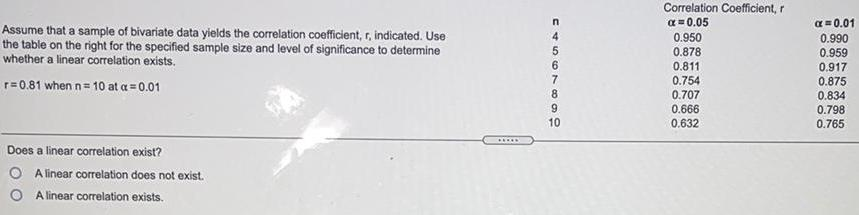Question:

# Assume that a sample of bivariate data yields the

Last updated: 7/23/2022Assume that a sample of bivariate data yields the correlation coefficient, r, indicated. Use the table on the right for the specified sample size and level of significance to determine whether a linear correlation exists. r=0.81 when n=10 at a = 0.01 Does a linear correlation exist? A linear correlation does not exist. OA linear correlation exists. C4567668 n 9 10 Correlation Coefficient, r α=0.05 0.950 0.878 0.811 0.754 0.707 0.666 0.632 α = 0.01 0.990 0.959 0.917 0.875 0.834 0.798 0.765